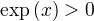A naive definition of exponentiation would be:But what happens when b is not a natural number?

Then, a better definition is:where "exp" is:where "ln" (the "natural logarithm") is:## Properties of "exp"

•••••• exp(x, x positive) rises very very quickly.

## Other Logarithmswhere:## Properties of (all) Logarithms

•(for positive x,y)
•(for positive x,y)
•